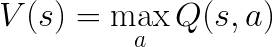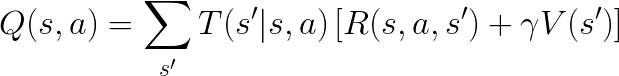# Tutorial: Creating a Planning and Learning Algorithm

## Tutorials (v1) > Creating a Planning and Learning Algorithm > Part 1

Tutorial Contents

You are viewing the tutorial for BURLAP 1; if you'd like the BURLAP 2 tutorial, go here.

### Introduction

In the previous tutorials, we walked through how you can use existing planning and learning algorithms in BURLAP on domains in BURLAP and how to create your own domains on which you can use those algorithms. However, you may also want to extend or create your own planning or learning algorithm in BURLAP that can either be used on existing domains or novel BURLAP domains and easily compared against existing algorithms. In this tutorial, we will show you how to create both a planning algorithm and a learning algorithm. In particular, we will be reimplementing versions of Value Iteration and Q-learning since they are conceptually simple algorithms to implement, but will expose the various properties of BURLAP you would want to use. In general, however, you should defer to using the existing implementations of these algorithms in BURLAP since they will support more features than we will cover here, such as support for planning and learning with Options (temporally extended actions). Using options in BURLAP will be left for a future tutorial.

### Value Iteration Overview

Value Iteration (VI) is an algorithm that finds the optimal value function (the expected discounted future reward of being in a state an behaving optimally from it), and consequentially the optimal policy, for an MDP's entire state space. Central to the idea of VI is the Bellman Equation, which states that the optimal value of a state is the value of the action with the maximum expected discounted future return (the action with the maximum Q-value) where the Q-value for a state-action pair is defined as the expected value over all possible state transitions of the immediate reward summed with the discounted value of the resulting state. In math:where T(s' | s, a) is the probability of transitioning to state s' when taking action a in state s, R(s, a, s') is the reward received for transitioning to state s' after taking action a in state s, and gamma is a discount factor affecting how much immediate rewards are preferred to later ones.

This equation presents some issues in that if an MDP has cycles, it's unclear what the values should be. However, the Value Iteration algorithm will converge to the optimal Value function if you simply initialize the value for each state to some arbitrary value, and then iteratively use the Bellman equation to update the the value for each state.

Planning algorithms that make use of the Bellman equation to estimate the Value function are known as Dynamic Programming (DP) planning algorithms. Different DP algorithms specify different priorities for when the estimated value of each state is updated with the Bellman equation. In the case of VI, Bellman updates are performed in entire sweeps of the state space. That is, at the start, the value for all states is initialized to some arbitrary value. Then, for each state in the state space, the Bellman equation is used to update the value function estimate. Sweeps over the entire state space are repeated for some fixed number of iterations or until the maximum change in the value function is small. The algorithm is summarized in the below pseudocode.

#### Value Iteration

1. Initialize value function V(s) arbitrarily for all states s.
2. Repeat until convergence...
3.     For each state s
4.         V(s) := max_a sum_s' T(s' | s, a) [R(s,a,s') + γ V(s')]

Since there are a number of different DP algorithms that can be implemented, BURLAP includes a macro abstract planning algorithm class called ValueFunctionPlanner that includes a number of helpful methods for automatically performing Bellman Updates on states. However, to give a better sense of how to use the more fundamental parts of a planning algorithm in BURLAP, we will instead write our VI algorithm from scratch. The only classes and interfaces we'll use are the OOMDPPlanner abstract class and the QComputablePlanner interface, which will set up a bunch of common data members for us and provide the common planning method interfaces that will let our planning algorithm implementation talk to other BURLAP tools.